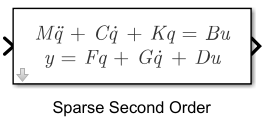# Sparse Second Order

Represent sparse second-order models in Simulink

• Library:
• Control System Toolbox

•## Description

The Sparse Second Order block lets you to represent second-order sparse state-space models, in Simulink®. Such sparse models arise from finite element analysis (FEA) and are useful in fields like structural analysis, fluid flow, heat transfer and electromagnetics. The resultant matrices from this type of modeling are quite large with a sparse pattern. In continuous time, a second-order sparse mass-spring-damper state-space model is represented in the following form:

Here, the full state vector is given by $\left[q,\stackrel{˙}{q}\right]$, where $q$ and $\stackrel{˙}{q}$ are the displacement and velocity vectors. `u` and `y` represent the inputs and outputs, respectively. `M`, `C`, and `K` represent the mass, damping and stiffness matrices, respectively. `B` is the input matrix, while `F` and `G` are the output matrices resulting from the two components of the state vector. `D` is the input-to-output matrix.

## Ports

### Input

expand all

Real-valued input vector of type `double` whose size is equal the number of columns in the B and D matrices.

Data Types: `double`

### Output

expand all

Real-valued output vector of type `double` whose size is equal to the number of rows in the F, G and D matrices.

Data Types: `double`

## Parameters

expand all

Mass matrix, specified as an `Nq`-by-`Nq` sparse matrix where, `Nq` is the number of nodes.

#### Programmatic Use

 Block Parameter: `M` Type: scalar, square sparse matrix Default: `1`

Damping matrix, specified as an `Nq`-by-`Nq` sparse matrix where, `Nq` is the number of nodes.

#### Programmatic Use

 Block Parameter: `C` Type: scalar, square sparse matrix Default: `0`

Stiffness matrix, specified as an `Nq`-by-`Nq` sparse matrix where, `Nq` is the number of nodes.

#### Programmatic Use

 Block Parameter: `K` Type: scalar, square sparse matrix Default: `1`

Input-to-state matrix, specified as an `Nq`-by-`Nu` sparse matrix where, `Nq` is the number of nodes and `Nu` is the number of inputs.

#### Programmatic Use

 Block Parameter: `B` Type: scalar, sparse matrix Default: `1`

Displacement-to-output matrix, specified as an `Ny`-by-`Nq` sparse matrix where, `Nq` is the number of nodes and `Ny` is the number of outputs.

#### Programmatic Use

 Block Parameter: `F` Type: scalar, sparse matrix Default: `1`

Velocity-to-output matrix, specified as an `Ny`-by-`Nq` sparse matrix where, `Nq` is the number of nodes and `Ny` is the number of outputs.

#### Programmatic Use

 Block Parameter: `G` Type: scalar, sparse matrix Default: `0`

Input-to-output matrix, specified as an `Ny`-by-`Nu` sparse matrix where, `Ny` is the number of outputs and `Nu` is the number of inputs.

#### Programmatic Use

 Block Parameter: `D` Type: scalar, sparse matrix Default: `0`

Initial values for displacement vector $q$, specified as a vector of doubles. $q$ and $\stackrel{˙}{q}$ are the displacement and velocity vectors that make up the state vector.

#### Programmatic Use

 Block Parameter: `q0` Type: scalar, vector of doubles Default: `0`

Initial values for velocity vector $\stackrel{˙}{q}$, specified as a vector of doubles. $q$ and $\stackrel{˙}{q}$ are the displacement and velocity vectors that make up the state vector.

#### Programmatic Use

 Block Parameter: `dq0` Type: scalar, vector of doubles Default: `0`

Note

For linearization with Simulink Control Design™, the linearized model is a `mechss` model object when the `Sparse Second Order` block is present in your Simulink model.

For an example, see Linearize Simulink Model to a Sparse Second-Order Model Object.